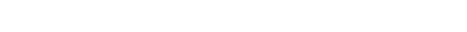October 16, 2023
October 16, 2023
October 16, 2023
###### Higher-Education-and-Politics
October 16, 2023
 Question 15
Consider the problem of reversing a singly linked list. To take an example, given the linked list below,Which one of the following statements is TRUE about the time complexity of algorithms that solve the above problem in O (1) space?

 ABCD It is not possible to reverse a singly linked list in O (1) space.
Question 15 Explanation:
struct Node {
int data;
struct Node* next;
{
this->data = data;
next = NULL;
}
};
/* Function to reverse the linked list */
void reverse()
{
// Initialize current, previous and
// next pointers
Node *prev = NULL, *next = NULL;
while (current != NULL) {
// Store next
next = current->next;
// Reverse current node’s pointer
current->next = prev;
// Move pointers one position ahead.
prev = current;
current = next;
}
}
For the above algorithm :
Time Complexity: O(n)
Space Complexity: O(1)
Question 15 Explanation:
struct Node {
int data;
struct Node* next;
{
this->data = data;
next = NULL;
}
};
/* Function to reverse the linked list */
void reverse()
{
// Initialize current, previous and
// next pointers
Node *prev = NULL, *next = NULL;
while (current != NULL) {
// Store next
next = current->next;
// Reverse current node’s pointer
current->next = prev;
// Move pointers one position ahead.
prev = current;
current = next;
}
}
For the above algorithm :
Time Complexity: O(n)
Space Complexity: O(1)